Get instant live expert help with Excel or Google Sheets“My Excelchat expert helped me in less than 20 minutes, saving me what would have been 5 hours of work!”

#### Post your problem and you’ll get expert help in seconds.

Your message must be at least 40 characters
Our professional experts are available now. Your privacy is guaranteed.

# Get last weekday in month

While planning activities and scheduling an event we often need to look for the date of a specific last weekday of the month in the calendar. In Excel, we can get the end of the month date for a given date using the EOMONTH function, and find the day of the week for a given date as a number between 1 to 7 using the WEEKDAY function. In this article, we will learn how to get the date of specific last weekday of the month in a formula based on EOMONTH and WEEKDAY functions.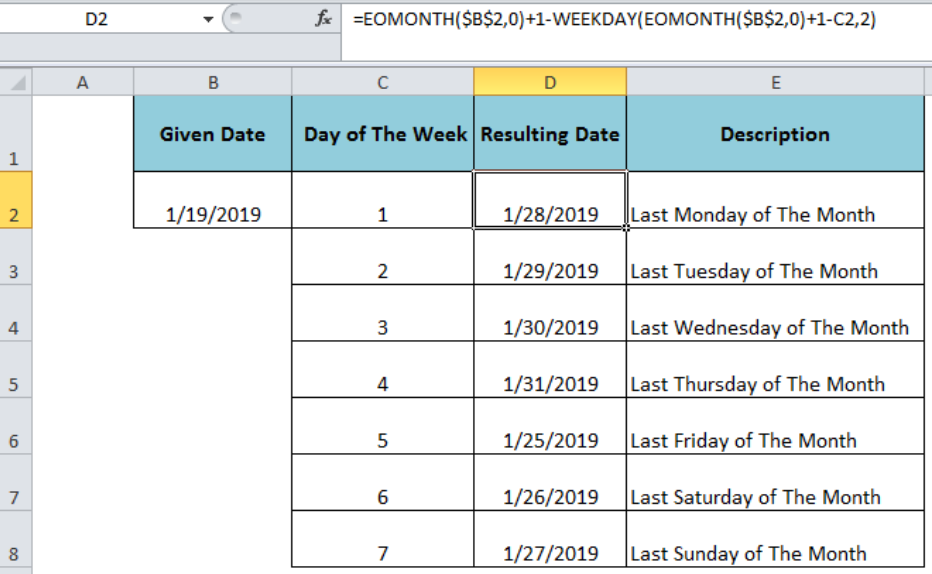Figure 1. Finding the Last Weekday of the Month

## Finding Date of The Specific Last Weekday of The Month

Suppose we have a given date in cell B2 and listed each day of the week as a number between 1 (Monday) and 7 (Sunday) in column C, and want to get the date of last weekday of the month, say last Monday of the month, in column D.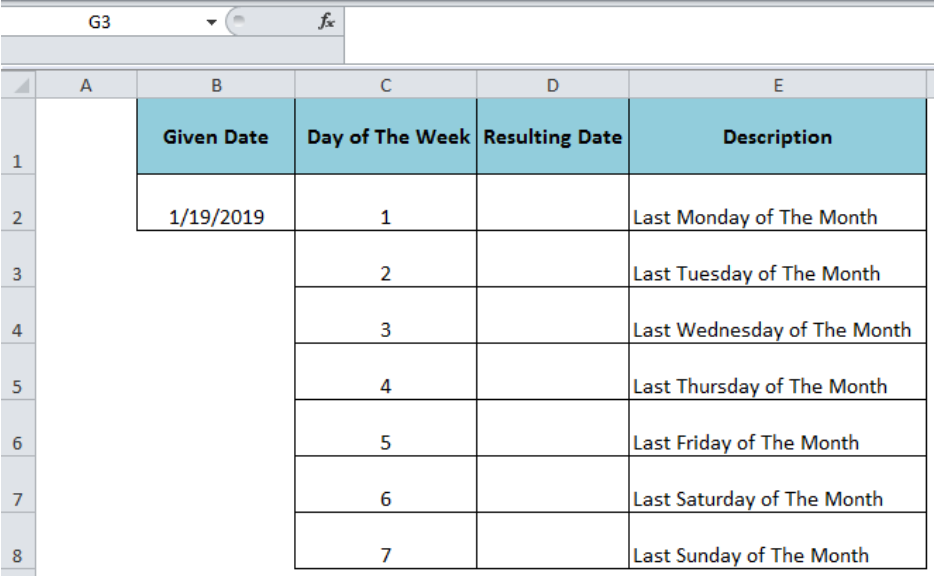Figure 2. Requested Last Days of The Week

The generic syntax of the formula would be;

`=EOMONTH(date,0)+1-WEEKDAY(EOMONTH(date,0)+1-day_number,2)`

To get the last Monday of the month in cell D2, format the cell D2 as date and insert the following formula in D2;

`=EOMONTH(\$B\$2,0)+1-WEEKDAY(EOMONTH(\$B\$2,0)+1-C2,2)`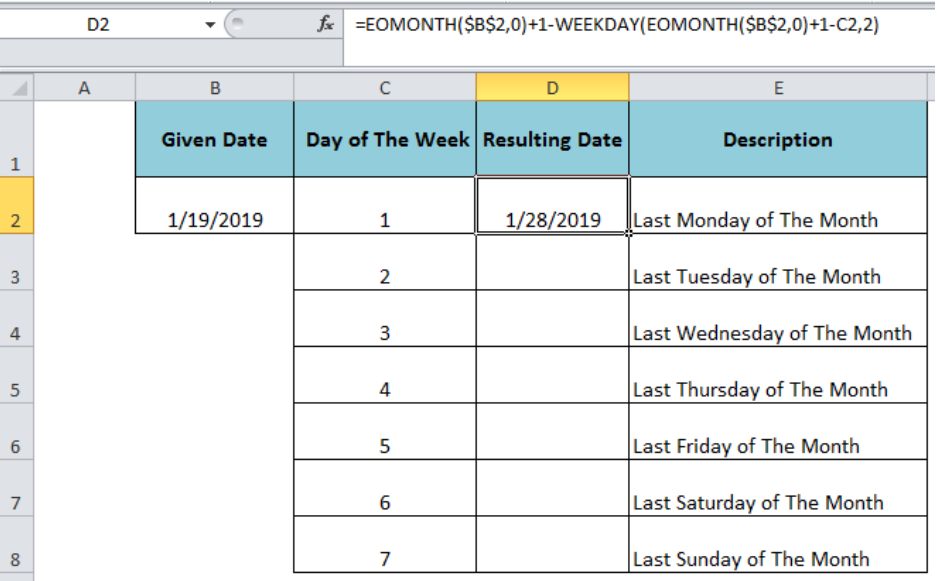Figure 3. Applying the Formula to Get Last Monday of the Month

The first part the formula =EOMONTH(\$B\$2,0)+1 returns the first day of next month, 2/1/2019 as per our example. In the next part, again the first day of next month is used and specific weekday number is subtracted from it, EOMONTH(\$B\$2,0)+1-C2. The resulting value is fed as the serial_number argument into the WEEKDAY function. As our weekday numbers are set from Monday to Sunday (1 to 7) so the return_type argument of the WEEKDAY function is set as 2 index number. Thus the WEEKDAY function returns a number (The Roll Back days), as given below;

`=WEEKDAY(EOMONTH(\$B\$2,0)+1-C2,2)`

`=4`

In the formula bar, select this part of the formula and press F9 to view the rollback days.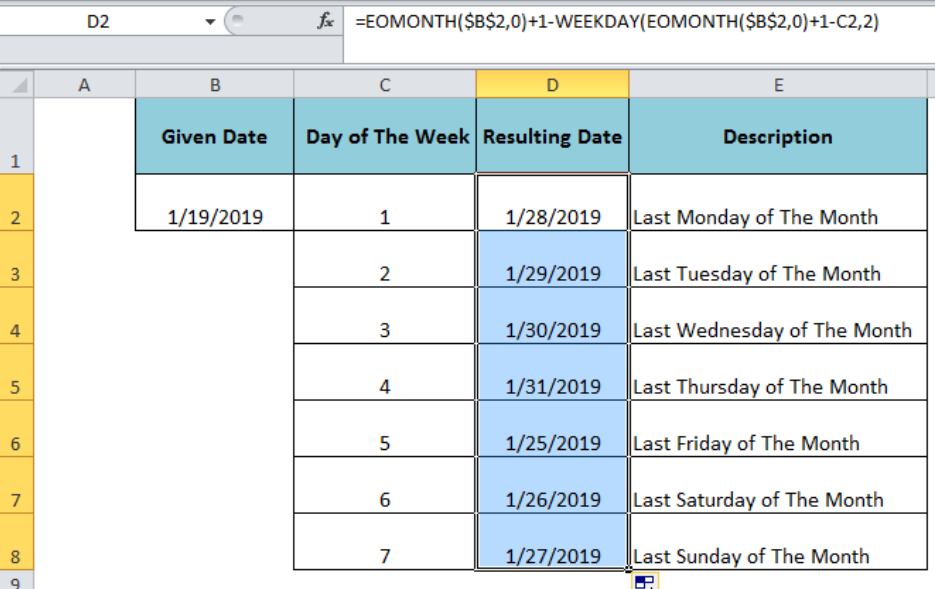Figure 4. Working of The Formula

This number is subtracted from the first part of the formula to roll back the first day of next month to get the desired last weekday of the month. Copy the formula to other cells to get the remaining resulting dates of the weekdays.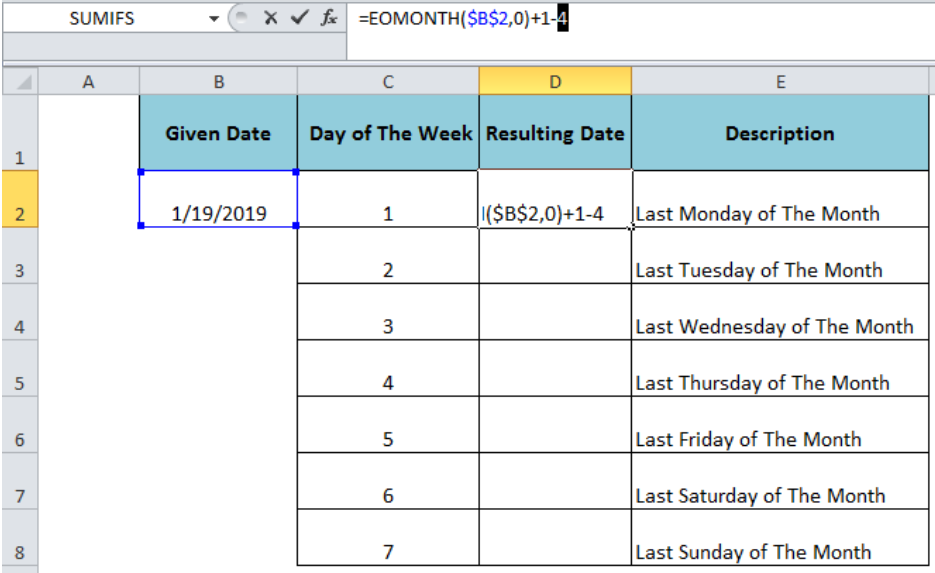Figure 4. Result For The Last Weekdays of The Month

## Instant Connection to an Expert through our Excelchat Service

Most of the time, the problem you will need to solve will be more complex than a simple application of a formula or function. If you want to save hours of research and frustration, try our live Excelchat service! Our Excel Experts are available 24/7 to answer any Excel question you may have. We guarantee a connection within 30 seconds and a customized solution within 20 minutes.

### Did this post not answer your question? Get a solution from connecting with the expert.Another blog reader asked this question today on Excelchat:
Solution examplesI need to sum the number of hours per month one of three halls are being rented
Solved by A. F. in 60 minsneed help with the different sheets and formula IF(A4=B4,0,NETWORKDAYS(A4+1,B4,\$E\$9:\$E\$42) +0.5*(INT((+B4-A4+1)/7)+IF(WEEKDAY(A4+1)+MOD (B4-A4-1,7)>=7,1,0)))
Solved by O. D. in 11 mins## Subscribe to Excelchat.coAnother blog reader asked this question today on Excelchat: